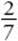﻿ ﻿CALCULATOR TIPS - Important Tactics - SAT SUBJECT TEST MATH LEVEL 1

## CHAPTER 1Important Tactics### CALCULATOR TIPS

You know, of course, that you are allowed to use a calculator whenever you like on the Math 1 test. (See SHOULD YOU USE A CALCULATOR ON THE MATH 1 TEST? for a discussion of calculator use.) The College Board classifies about 10 of the 50 questions on the Math 1 test as “calculator active.” For these questions, a calculator is absolutely required. No one can evaluate tan 23°oror 2125 without a calculator. Another 10 to 15 questions are classified as “calculator neutral.” On those questions, the use of a calculator is optional. You can surely evaluate 27 ororof 168 or 987 − 789 without a calculator, but why should you? You have a calculator, so use it.

If there is any chance that you will make a mistake adding or subtracting negative numbers, use your calculator. A general rule of thumb is this: do not do arithmetic in your exam booklet. If you cannot do it in your head, use your calculator. In particular, never do long multiplication or long division; do not find common denominators; do not simplify radicals; do not rationalize denominators. All these things you should do on your calculator.

THROUGHOUT THIS BOOK, WHENEVER THE USE OF A CALCULATOR IS REQUIRED OR RECOMMENDED, A CALCULATOR ICONAPPEARS IN THE MARGIN.

The College Board considers the other 25 to 30 questions on the Math 1 test to be “calculator inactive.” These are questions about which they say, “There is no advantage, perhaps even a disadvantage, to using a calculator.” The discussion under TACTIC 1, shows you that even on some of these calculator inactive questions, if you get stuck, there is a way to use your calculator to get the right answer.

﻿